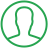# Count Vowels In A String Python

Last Updated : Jul 1, 2023In this article we will show you the solution of count vowels in a string python, programming relies heavily on strings.

The number of vowel in a string must be calculated taking into account both upper case and lower case letters because programming languages are typically case sensitive.

The most fundamental method you will use to solve an issue is the brute force method.

The loop only executes n times because the string's length is the only length for which it can run.

As a result, the brute force approach's Time Complexity is O. (n). We'll now talk about the idea of counting vowel in a string of Python.

## Step By Step Guide On Count Vowels In A String Python :-

### Method - Brute Force Approach

```ex = "Count number of vowels in a String in Python"
count = 0
i = 0
for i in range(len(ex)):
if (
(ex[i] == "a")
or (ex[i] == "e")
or (ex[i] == "i")
or (ex[i] == "o")
or (ex[i] == "u")
):
count += 1
print("Number of vowel in the string is: ", count)```
1. Here, you are able to observe that we use a brute-force strategy to construct a Python code for determining the amount of vowel in a string.
2. We initialise the string at the beginning of our code. We then set a count variable's initial value to 0.
3. It then makes use of a stated index variable. The string's characters should all be handled equally.
4. Next, we apply a for loop to Here, a value of I is increased by 1 and checked to ensure that it is between 0 as well as the string's length using the range() method.
5. A vowel is a letter, thus check "IF" it is a vowel after that. Add a single increment to the count variable's value if a character is a vowel.
6. The string is then iterated over using Else continue. When the string runs out, cut the loop.
7. The final step uses Python's print function to display a number of vowel in a string.

### Method - using 'in' operator:

```ex = "Count the number of vowel in a String in Python"
count = 0
vowels = ["a", "e", "i", "o", "u"]
for i in range(len(ex)):
if ex[i] in vowels:
count += 1
print("Number of vowels in the given string is: ", count)```
1. We can see that we use the in operator to build the Python code in this example to determine the number of vowel in a string.
2. The "in" operator in the Python programming language determines if a value is available in the provided sequence.
3. We begin by setting the count variable and the string's initial value.
4. Next, a list including the vowels was made. You can also include the uppercase vowel characters in the list in this fashion.
5. We then use len, a Python function that returns the string's total length, to iterate over the supplied string.
6. Once the character is determined to be included in the list containing vowels, the if statement is used to determine its presence.
7. When a vowel is present, this produces the word "Yes"; otherwise, it produces the word "False".
8. The final line of our code uses Python's print function to print the outcome.

## Conclusion :-

As a result, we have learned valuable how to count vowel in a Python string.

We also discovered that applying raw force is the most basic approach you will take to problem-solving.

The length of the string represents the maximum length for which the loop can run, therefore it only runs n times.

I hope this article on count vowels in a string python helps you and the steps and method mentioned above are easy to follow and implement.

##About Ashish

Ashish is a dynamic and motivated individual with a passion of programming and an experienced programmer having 3+ years of experience in various languages like Java, Python, HTML, CSS, JavaScript, jQuery and various frameworks like Bootstrap